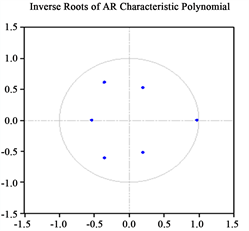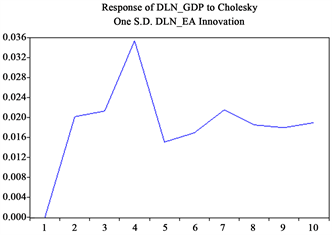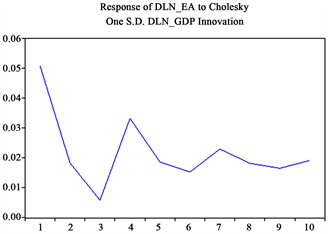基于VECM的教育投资与经济增长关系研究Research on the Relationship between Education Investment and Economic Growth Based on VECM

• 全文下载: PDF(741KB)    PP.248-254   DOI: 10.12677/FIN.2018.86029
• 下载量: 183  浏览量: 557   科研立项经费支持

As a part of human capital, the increase of education investment in expenditure will directly affect the development of the entire social economy. Similarly, the economy, as a material basis for in-creased investment in education, plays an important role in enhancing the investment capacity of education in all aspects of society. Taking China’s 1985-2017 as an example, this paper empirically analyzes the relationship between education investment and economic growth by establishing VAR and VEC models, using Johansen cointegration analysis and impulse response methods. The results show that education investment plays a greater role in promoting economic growth than the role of economic growth playing in education investment in China, and economic growth has a certain time lag in promoting education investment.

1. 引言

2. 相关文献综述

3. 模型构建和统计分析

3.1. 平稳性检验

3.2. VAR模型建立及最优滞后阶数确定

VAR (Vector Auto Regressive)模型由Sims (1980)提出，是当今世界上分析经济系统动态性的主流模型之一。VAR模型可以表述如下：

${Y}_{t}={\alpha }_{1}{Y}_{t-1}+{\alpha }_{2}{Y}_{t-2}+\cdots +{\alpha }_{p}{Y}_{t-p}+{\epsilon }_{t}$ (1)

${Y}_{t}=\left[\begin{array}{cc}-0.143& -0.011\\ 0.568& 0.498\end{array}\right]\ast {Y}_{t-1}+\left[\begin{array}{cc}-0.163& -0.126\\ 0.461& 0.551\end{array}\right]\ast {Y}_{t-2}+{\epsilon }_{t}$ (2)Table 2. Optimal lag order determination

${Y}_{t}=\left[\begin{array}{cc}-0.112& 0.029\\ 0.334& 0.254\end{array}\right]\ast {Y}_{t-1}+\left[\begin{array}{cc}-0.140& -0.055\\ 0.305& 0.228\end{array}\right]\ast {Y}_{t-2}+\left[\begin{array}{cc}-0.152& 0.020\\ 0.494& 0.466\end{array}\right]\ast {Y}_{t-3}+{\epsilon }_{t}$ (3)

3.3. Johansen协整检验及VEC模型建立Table 3. Johansen cointegration test process

${\text{DLN_GDP}}_{t-1}=0.223{\text{DLN_EA}}_{t-1}+0.114$ (4)

$\begin{array}{l}\Delta D\left({\text{DLN_GDP}}_{t}\right)\\ =-1.623{\text{ECM}}_{t-1}+0.407D\left({\text{DLN_GDP}}_{t-1}\right)+0.200D\left({\text{DLN_GDP}}_{t-2}\right)\\ \text{\hspace{0.17em}}\text{\hspace{0.17em}}\text{ }-0.221D\left({\text{DLN_EA}}_{t-1}\right)-0.155D\left({\text{DLN_EA}}_{t-2}\right)-0.003\end{array}$ (5)

$\begin{array}{l}\Delta D\left({\text{DLN_EA}}_{t}\right)\\ =-0.108{\text{ECM}}_{t-1}+0.102D\left({\text{DLN_GDP}}_{t-1}\right)+0.011D\left({\text{DLN_GDP}}_{t-2}\right)\\ \text{\hspace{0.17em}}\text{\hspace{0.17em}}\text{ }-0.740D\left({\text{DLN_EA}}_{t-1}\right)-0.477D\left({\text{DLN_EA}}_{t-2}\right)-0.005\end{array}$ (6)

3.4. 脉冲响应分析Figure 1. AR root test resultsFigure 2. Impulse response of DLN_EA to DLN_GDPFigure 3. Impulse response of DLN_ GDP to DLN_EA

4. 结束语